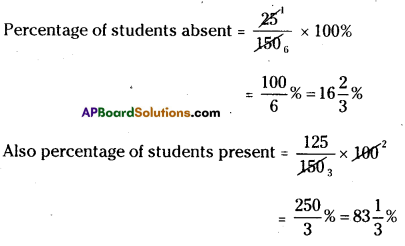# AP Board 7th Class Maths Solutions Chapter 6 Ratio – Applications Ex 4

AP State Syllabus AP Board 7th Class Maths Solutions Chapter 6 Ratio – Applications Ex 4 Textbook Questions and Answers.

## AP State Syllabus 7th Class Maths Solutions 6th Lesson Ratio – Applications Exercise 4Question 1.
In a school X, 48 students appeared for 10th class exam out of which 36 students passed. In another school Y, 30 students appeared and 24 students passed. If the District Educational Officer wants to give an award on the basis of pass percentage. To which school will he give the award?
Solution:
From the first school:
Number of students appeared for the exam = 48
Number of students passed = 36
as a percentage = $$\frac { 36 }{ 48 }$$ × 100 = 75
Pass percentage = 75%

From the second school:
Number of students appeared = 30
Number of students passed = 24
Pass percentage = $$\frac { 24 }{ 30 }$$ × 100 = 80%
As 80% > 75%
D.E.O should give award to the 2nd school.Question 2.
Last year the cost of 1000 articles was ₹ 5000. This year it goes down to ₹ 4000. What is the percentage of decrease in price?
Solution:
Last year
Cost of 1000 articles = ₹ 5000
∴ Cost of one article = $$\frac { 5000 }{ 1000 }$$ = ₹ 5
This year
Cost of 1000 articles = ₹ 4000
∴ Cost of one article = $$\frac { 4000 }{ 1000 }$$ = ₹ 4
Decrease in price = ₹ 5 – ₹ 4 = ₹ 1
Decrease In percentage = $$\frac { 1 }{ 5 }$$ × 100% = 20%

Question 3.
Sri Jyothi has a basket full of bananas, oranges and mangoes. If 50% are bananas, 15% are oranges, then what percent are mangoes?
Solution:
Percent of bananas = 50%
Percent of oranges = 15%
∴ Percent of mangoes = 100% – (50% + 15%)
= 100% – 65% = 35%Question 4.
64% + 20% + …..? = 100%
Solution:
(64% + 20%) +…………… = 100%
∴ ?=100% – 84% = 16%

Question 5.
On a rainy day, out of 150 students in a school 25 were absent. Find the percentage of students absent from the school? What percentage of students are present?
Solution:
Total students = 150
Number of students absent = 25
∴ Number of students present = 150 – 25 = 125Question 6.
Out of 12000 voters in a constituency, 60% voted. Find hte number of people voted in the
constituency?
Solution:
Percentage of people who voted = 60% of I 2,000
∴ Number of people voted = $$\frac{60 \times 12000}{100}$$ = 7,200Question 7.
A local cricket team played 20 matches in one season. If it won 25% of them and lost rest. How many matches did it loose?
Solution:
Total number of matches played = 20
Matches won = 25%
∴ Matches lost = (100 –  25)% of total played
= 75% of 20
$$\frac{75 \times 20}{100}$$ = 15

Question 8.
In every gram of gold, a goldsmith mixes 0.25 grams of silver and 0.05 grams of copper. What is the percentage of gold, silver and copper in every gram of gold?
Solution:
In 1 gm
The quantity of silver = 0.25 gm
The quantity of copper = 0.05 gm
∴ The quantity of gold = 1 gm – (0.25 . 0.05)
= 1 – 0.3 = 0.7gm
∴ Percentage of gold = $$\frac { 0.7 }{ 1 }$$ × 100 = 70%
∴ Percentage of silver = $$\frac { 0.25 }{ 1 }$$ × 100 = 25%
∴ Percentage of copper = $$\frac { 0.05 }{ 2 }$$ × 100 = 5%Question 9.
40% of a number is 800 then find the number?
Solution:
Let the number be = x
40% of x = 800
(i.e.,) $$\frac{40 \times x}{100}$$ = 800
x = $$\frac{800 \times 100}{40}$$
x = 2000
∴ The required number = 2000